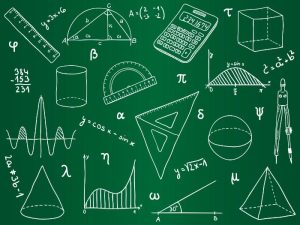## The Definition of Statistics in Math

Statistics in math is the study of data and their interpretation. It is the science of collecting and analyzing data, summarizing them, and presenting them in a way that can be easily understood by others. The field is a branch of mathematics that is used in a wide variety of scientific disciplines, including the physical and social sciences.It also is a key component of many academic programs in business and the humanities. In addition to providing information for scientific purposes, statistics can be used to forecast future occurrences.

The term “statistics” comes from the Latin stat-teres, which means “to gather” or “to record.” It is the study of the numbers and patterns that result when information is gathered, summarized, and analyzed.

Typically, statisticians collect data using three basic methods: surveys, experiments, and reliable sources. The data they collect can then be analyzed to create the mathematically defined “numbers” that are called statistics.

A statistic is a numerical value derived from the mathematical analysis of a dataset that either describes features of that set or makes inferences and predictions based on those patterns. The most common types of statistics are descriptive and inferential.

Descriptive statistics are most often concerned with two sets of properties of a distribution (sample or population): central tendency and dispersion. Central tendency seeks to describe the central or typical value of the distribution, while dispersion characterizes the extent to which members of the distribution vary from this central value.

Inferential statistics, on the other hand, use statistical techniques to make generalizations about populations based on sample characteristics. They analyze the central tendency, variability, and distribution of a data sample to identify trends that may indicate relevant characteristics in large populations.

Skewness, or asymmetricity, is a property of the distribution of a set of data. It is a measure of how far outward the values from the mean and standard deviation vary from one another. It can be positive, negative, or zero.

The statistics field also encompasses the mathematical theory of probability. Probability theories can be used to estimate how likely an event is to occur or to calculate the odds that two events will happen at the same time.

These concepts are important to understand how statistics work and why they are useful. They can help you to understand how to gather, interpret, and use data in ways that are both logical and effective.

Statistics is a complex field of research and analysis. It requires a combination of mathematical skills and the ability to think critically.

It can be a difficult subject to master, but it is essential for students of all levels. It will enable them to evaluate data and make informed decisions about their lives, careers, and the world around them.

Statistics is a subset of math that focuses on applied mathematics. While some mathematicians like to think of statistics as a broader discipline that is separate from math, most students see it as a complement and an extension of the more abstract aspects of math.

In conclusion, statistics in math is a discipline that revolves around the study of data, its interpretation, and the mathematical analysis used to derive meaningful information from it. It is a vital tool in various scientific fields, as well as in business and the humanities.

The term “statistics” stems from the Latin word “stat-teres,” which emphasizes the act of gathering and recording information. Statisticians employ various methods such as surveys, experiments, and reliable sources to collect data, which is then analyzed and summarized into numerical values known as statistics.

Statistics can be broadly categorized into descriptive and inferential statistics. Descriptive statistics focus on the properties of a data distribution, including central tendency and dispersion, while inferential statistics make generalizations and predictions about populations based on sample characteristics.

Additionally, the field of statistics incorporates probability theory, which enables the estimation of event likelihoods and the calculation of joint probabilities. Understanding these concepts is crucial for effectively gathering, interpreting, and utilizing data in logical and meaningful ways.

Mastering statistics requires a combination of mathematical skills and critical thinking abilities. While it may pose challenges, acquiring proficiency in statistics empowers individuals to evaluate data, make informed decisions, and comprehend the world around them.

Statistics is often viewed as an applied branch of mathematics, complementing the more abstract aspects of the subject. It plays a fundamental role in shaping our understanding of the world, providing tools for analysis, decision-making, and forecasting.

In summary, statistics in math offers valuable insights into data analysis, interpretation, and prediction. It is a powerful discipline that equips individuals with the skills to navigate and comprehend complex information, enabling informed decision-making in various fields of study and practice.